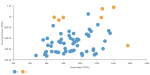# DCPY.LOFOUTLIER(n_neighbors, leaf_size, contamination, columns)

LOF (Local Outlier Factor) is an unsupervised outlier detection method that computes local density deviations of a given data point comparing to its neighbors. Locality is given by K-nearest neighbors, whose distance is used to estimate the local density. By comparing densities, the algorithm identifies samples with significantly lower density than its neighbors, which are considered as outliers. For measuring the distance, Minkowski metric is used.

###### Parameters
• n_neighbors – Number of neighbors considered for locality. Choose a number that is greater than minimum data points that a cluster of dense data points should contain, integer (default 20).
• leaf_size – Controls the size of the tree constructed in K-nearest neighbor calculations. It affects the speed and memory consumption of the queries, integer (default 30).
• contamination – Approximate proportion of outliers in the data set, which is used as a threshold for the decision function, float (0;1), (default 0.1).
• columns – Dataset columns or custom calculations.

Example: DCPY.LOFOUTLIER(20, 30, 0.1, sum([Gross Sales]), sum([No of customers])) used as calculation for the Color field of the Scatterplot visualization.###### Input data
• Numeric variables are automatically scaled to zero mean and unit variance.
• Character variables are transformed to numeric values using one-hot encoding.
• Dates are treated as character variables, so they are also one-hot encoded.
• Size of input data is not limited, but many categories in character or date variables rapidly increase the dimensionality.
• Rows that contain missing values in any of their columns are dropped.
###### Result
• Column of values 1 correspond to inlier, and -1 correspond to outlier.

• Rows that were dropped from input data due to containing missing values have missing values instead of assigned inlier/outlier values.

###### Key usage points
• Local approach, which is able to identify outliers in a data set that would not be outliers in another area of the data set.
• Suitable also for big data sets.
• Unpredictable impact of n_neighbors parameter on identifying outliers
###### Example

For the whole list of algorithms, see Data science built-in algorithms.